Courses
Courses for Kids
Free study material
Offline Centres
More

# Perimeter and Area Class 7 Notes CBSE Maths Chapter 11 (Free PDF Download)Last updated date: 02nd Dec 2023
Total views: 593.4k
Views today: 14.93k

## Revision Notes for CBSE Class 7 Maths Chapter 11 - Free PDF Download

Free PDF download of Class 7 Maths Chapter 11 - Perimeter and Area Revision Notes & Short Key-notes prepared by expert Maths teachers from latest edition of CBSE(NCERT) books. To register Maths Tuitions on Vedantu.com to clear your doubts. You can also register Online for NCERT Solutions Class 7 Science tuition on Vedantu.com to score more marks in CBSE board examination. Vedantu is a platform that provides free CBSE Solutions (NCERT) and other study materials for students. Maths Students who are looking for the better solutions, they can download Class 7 Maths NCERT Solutions to help you to revise complete syllabus and score more marks in your examinations.

Also, check CBSE Class 7 Maths revision notes for All chapters:

## Access Class 7 Mathematics Chapter 11 - Perimeter and Area Notes

• The area of a closed figure is the part of the plane occupied by the closed-form, whereas the perimeter is the distance surrounding it.

• The area of a plane or region enclosed by it is measured in square metres.

• In a previous lecture, we learned how to calculate the perimeter and area of a square and rectangle. They are as follows:

• Perimeter of a square $\text{= 4 }\!\!\times\!\!\text{ side}$

• Perimeter of a rectangle $\text{= 2 }\!\!\times\!\!\text{ }\left( length+breadth \right)$

• Area of a square $\text{= side }\!\!\times\!\!\text{ side}$

• Area of a rectangle $\text{= length }\!\!\times\!\!\text{ breadth}$

Example:

1. A wire is in the shape of a square of side $\text{12 cm}$. If the wire is rebent into a rectangle of length $\text{16 cm}$, find its breadth. Which encloses more area, the square or the rectangle?

Ans:Side of the Square $\text{12 cm}$

Here,

Length of the wire $\text{=}$ Perimeter of the square

Therefore,

$\text{Length of the wire = 4 }\!\!\times\!\!\text{ side}$

$\text{Length of the wire = 4 }\times \text{ 12 cm}$

$\text{Length of the wire = 48 cm}$

Therefore, length of the wire is $\text{48 cm}$.

We have given, the Length of the rectangle.

Let’s, consider $\text{l}$ be the length of the rectangle and $\text{b}$ be the breadth of the rectangle.

Now,

Perimeter of rectangle $\text{=}$ Length of wire

Hence,

$\text{Perimeter of rectangle = 48 cm ---}\left( 1 \right)$

Since,

Perimeter of a rectangle $\text{= 2 }\!\!\times\!\!\text{ }\left( length+breadth \right)$

Eq, $\left( 1 \right)$ becomes,

$\text{ 48 = 2 }\!\!\times\!\!\text{ }\left( \text{16 + b} \right)$

$\dfrac{\text{48}}{\text{2}}\text{ = 16 + b}$

$\text{ 24 = 16 + b}$

$\text{ b = 24 - 16}$

Therefore,

$\text{ b = 8 cm }$

Hence, breadth of the rectangle is $\text{8 cm }$.

Area of a square $\text{= }{{\left( \text{side} \right)}^{2}}$

Therefore,

$\text{Area of a square = }{{\left( \text{side} \right)}^{\text{2}}}$

$\text{ = }{{\left( \text{12} \right)}^{\text{2}}}$

$\text{ = 12 }\!\!\times\!\!\text{ 12}$

$\text{Area of a square = 144 c}{{\text{m}}^{\text{2}}}$

$\text{Area of a rectangle = }\left( \text{l }\!\!\times\!\!\text{ b} \right)$

$\text{ = }\left( \text{16 }\!\!\times\!\!\text{ 8} \right)$

$\text{Area of a rectangle = 128 c}{{\text{m}}^{\text{2}}}$

Therefore, the square encloses more area even though its perimeter is the same as that of the rectangle.

• Triangles as Parts of Rectangles:

• The area of the rectangle is equal to the sum of the two triangles' areas.

• The area of both triangles is the same.• For above rectangle,

$\text{Area of each triangle = }\dfrac{\text{1}}{\text{2}}\left( \text{Area of the rectangle} \right)$

$\text{Area of each triangle = }\dfrac{\text{1}}{\text{2}}\times \text{ l }\times \text{ b}$

• For below square,$\text{Area of each triangle = }\dfrac{\text{1}}{4}\left( \text{Area of the square} \right)$

$\text{Area of each triangle = }\dfrac{\text{1}}{\text{4}}{{\left( side \right)}^{2}}$

Example: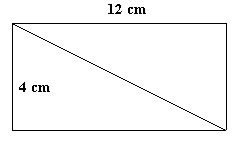Ans:

$\text{Area of each triangle = }\dfrac{\text{1}}{\text{2}}\times \text{ l }\times \text{ b}$

$\text{Area of each triangle = }\dfrac{\text{1}}{\text{2}}\times \text{ 12 }\times \text{ 4}$

$\text{Area of each triangle = 24 c}{{\text{m}}^{2}}$

• Area of a Parallelogram:

• Any side of a parallelogram can be considered as the base of the parallelogram.

• The perpendicular drawn on that side from the opposite vertex is known as height (altitude).

• $\text{Area of a Parallelogram = Base }\!\!\times\!\!\text{ Height}$

Example:

1. One of the sides and the corresponding height of a Parallelogram are $\text{17 cm}$ and $\text{7 cm}$ respectively. Find the area of the parallelogram.

Ans: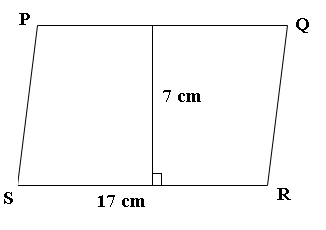Here, Base of Parallelogram is, $\text{b = 17 cm}$

and, Height of Parallelogram is $\text{h = 7 cm}$

Therefore,

$\text{Area of a Parallelogram = Base }\!\!\times\!\!\text{ Height}$

$\text{ = 17 }\!\!\times\!\!\text{ 7}$

$\text{Area of a Parallelogram = 144 }{{\text{m}}^{\text{2}}}$

Therefore, area of a Parallelogram is $\text{144 }{{\text{m}}^{\text{2}}}$.

• Area of a Triangle:

• Area of a triangle  $\text{= }\dfrac{1}{2}\left( \text{Area of the parallelogram generated from it} \right)$

Therefore,

$\text{Area of a triangle = }\dfrac{\text{1}}{\text{2}}\text{ }\!\!\times\!\!\text{ Base }\!\!\times\!\!\text{ Height}$

• $\text{Area of an equilateral triangle = }\dfrac{\sqrt{3}}{4}\text{ }{{\left( side \right)}^{2}}$

• All congruent triangles have the same area, however triangles with the same area do not have to be congruent.

Example:

Find the area of the given triangle.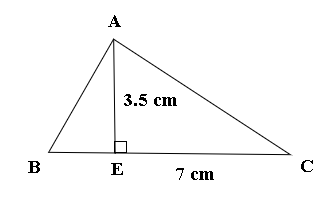Ans:

Here, Base of triangle is $\text{b = 7 cm}$

and, Height of triangle is $\text{h = 3}\text{.5 cm}$

Therefore,

$\text{Area of a triangle = }\dfrac{\text{1}}{\text{2}}\text{ }\!\!\times\!\!\text{ Base }\!\!\times\!\!\text{ Height}$

$\text{Area of a triangle = }\dfrac{\text{1}}{\text{2}}\text{ }\!\!\times\!\!\text{ B }\!\!\times\!\!\text{ H}$

$\text{ = }\dfrac{\text{1}}{\text{2}}\text{ }\!\!\times\!\!\text{ 7 }\!\!\times\!\!\text{ 3}\text{.5}$

$\text{Area of a triangle = 12}\text{.25 c}{{\text{m}}^{\text{2}}}$

Therefore, area of a triangle is $\text{12}\text{.25 c}{{\text{m}}^{\text{2}}}$.

• Circumference of a Circle:

• The circumference of a circular location is the distance around it.

• The ratio of a circle's circumference to diameter of a circle is constant and denoted by $\pi \text{ }\left( pi \right)$.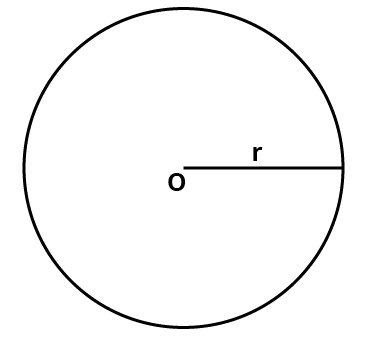• Circumference of a circle in terms of diameter:

$\text{Circumference of a circle }\left( C \right)\text{ = }\pi \text{d}$

Where, $\text{d}$ is the diameter of a circle.

$\text{ }\!\!\pi\!\!\text{ = }\dfrac{\text{22}}{\text{7}}$

$\text{ }\!\!\pi\!\!\text{ = 3}\text{.14 (Approx}\text{.)}$

• Circumference of a circle in terms of radius:

$\text{Circumference of a circle }\left( \text{C} \right)\text{ = }\!\!\pi\!\!\text{ d}$

$\text{Circumference of a circle }\left( \text{C} \right)\text{ = }\!\!\pi\!\!\text{ }\!\!\times\!\!\text{ 2r}$

$\text{Circumference of a circle }\left( \text{C} \right)\text{ = 2 }\!\!\pi\!\!\text{ r}$

Example:

1. What is the circumference of a circular disc of radius $\text{21 cm}$?

Ans:

Here, radius of a circular disc is $\text{r = 21 cm}$

Thus, circumference of a disc,

$\text{C = 2 }\!\!\pi\!\!\text{ r}$

$\text{C = 2 }\!\!\times\!\!\text{ }\dfrac{\text{22}}{\text{7}}\text{ }\!\!\times\!\!\text{ 21}$

$\text{C = 132 cm}$

Therefore, the circumference of a circular disc is $\text{132 cm}$.

• Area of Circle:

$\text{Area of a Circle = }\!\!\pi\!\!\text{ }{{\text{r}}^{\text{2}}}$

Where, $\text{r}$ is the radius of the circle.

Example:

1. Diameter of a circular garden is $\text{10}\text{.6 m}$. Find its area.

Ans: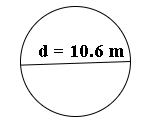Diameter of a given circular garden is

$\text{d = 10}\text{.6 m}$

Therefore, the radius of a circle is

$\text{r = }\dfrac{d}{2}$

$\text{r = }\dfrac{\text{10}\text{.6}}{2}$

$\text{r = 5}\text{.3 m}$

Thus,

$\text{Area of a Circle = }\!\!\pi\!\!\text{ }{{\text{r}}^{\text{2}}}$

$\text{Area of a Circle = }\dfrac{\text{22}}{\text{7}}{{\left( \text{5}\text{.3} \right)}^{\text{2}}}$

$\text{Area of a Circle = }\dfrac{\text{22}}{\text{7}}\text{ }\!\!\times\!\!\text{ 5}\text{.3 }\!\!\times\!\!\text{ 5}\text{.3}$

$\text{Area of a Circle = 88}\text{.28 }{{\text{m}}^{\text{2}}}$

Therefore, area of a circular garden is $\text{88}\text{.28 }{{\text{m}}^{\text{2}}}$.

• Conversion of Units:

• The units of area can be translated using the same method as the units of lengths that we learned earlier:

• $\text{ 1 c}{{\text{m}}^{\text{2}}}\text{ = 100 m}{{\text{m}}^{\text{2}}}$

$\text{ 1 }{{\text{m}}^{\text{2}}}\text{ = 10000 c}{{\text{m}}^{\text{2}}}$

$\text{1 hectare = 10000 }{{\text{m}}^{\text{2}}}$

• The number of units produced by converting a unit of area to a smaller unit will be greater.

Example:

$\text{10000 c}{{\text{m}}^{\text{2}}}\text{ = 10000 }\!\!\times\!\!\text{ 100 m}{{\text{m}}^{\text{2}}}$

$\text{10000 c}{{\text{m}}^{\text{2}}}\text{ = 1000000 m}{{\text{m}}^{\text{2}}}$

• The number of larger units will be smaller when we convert a unit of area to a larger unit.

$\text{10000 c}{{\text{m}}^{\text{2}}}\text{ = }\dfrac{\text{10000}}{10000}\text{ }{{\text{m}}^{\text{2}}}$

$\text{10000 c}{{\text{m}}^{\text{2}}}\text{ = 1 }{{\text{m}}^{\text{2}}}$

Example:

1. A rectangular park is $\text{55 m}$ long and $\text{20 m}$ wide. A path $\text{3}\text{.5 m}$ wide is constructed outside the park. Find the area of the path.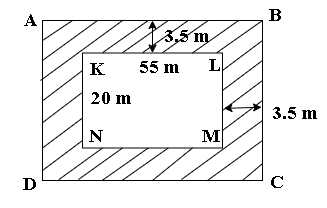Consider $\text{KLMN}$ rectangle which represent the rectangular park and the shaded region represent the path $\text{3}\text{.5 m}$ wide.

To find the area of the path, first we need to find $\left( \text{Area of rectangle ABCD - Area of rectangle KLMN} \right)$

Now,

$\text{AB = 3}\text{.5 + 55 + 3}\text{.5}$

$\text{AB = 62 m}$

$\text{AD = 3}\text{.5 + 20 + 3}\text{.5}$

$\text{AD = 27 m}$

$\text{Area of rectangle ABCD = l }\times \text{ b}$

$\text{Area of rectangle ABCD = AB }\times \text{ AD}$

$\text{Area of rectangle ABCD = 62 }\times \text{ 27}$

Therefore,

$\text{Area of rectangle ABCD = 1,674 }{{\text{m}}^{2}}$

$\text{Area of rectangle KLMN = l }\times \text{ b}$

$\text{Area of rectangle KLMN = KL }\times \text{ KN}$

$\text{Area of rectangle KLMN = 55 }\times \text{ 20}$

Hence,

$\text{Area of rectangle KLMN = 1,100 }{{\text{m}}^{2}}$

Thus,

$\text{Area of Path = }\left( \text{Area of rectangle ABCD - Area of rectangle KLMN} \right)$

$\text{Area of Path = 1674 - 1100}$

Therefore,

$\text{Area of Path = 574 }{{\text{m}}^{2}}$.

## FAQs on Perimeter and Area Class 7 Notes CBSE Maths Chapter 11 (Free PDF Download)

Q1. Where can I download the latest Revision Notes of Chapter 11 of Class 7 Maths?

Ans: You can download the latest Revision Notes of Chapter 11 of Class 7 Maths from the official website of Vedantu. It has all the notes of the chapters included in Class 7. They are well-curated and comprehensive. You will find important questions in those notes and it will be easier for you to understand the basics from those notes. You can use those notes a day before your exam to make revision easy.  The notes are free of cost. They are also available on vedantu Mobile app.

Q2. How the concept of geometry with respect to the chapter is included in NCERT Solutions?

Ans:Geometry is a discipline that entails a thorough examination of all the figures that surround us, with a focus on determining the area and perimeter of those figures. Because of their importance in our daily lives, these factors must be calculated. As a result, the NCERT solutions for class 7 maths chapter 11 are available. The theories of area and perimeter of various forms such as the square, rectangle, parallelogram, triangle, and circle are covered in Perimeter and Area. When it comes to the area and perimeter of shapes, unit understanding is equally critical; so, the NCERT solutions class 7 mathematics chapter 11 The conversion of lengths and areas into dimensions is also explained by perimeter and area.

Q3. Shazli took a wire of length 44 cm and bent it into the shape of a circle. Find the radius of that circle. Also, find its area. If the same wire is bent into the shape of a square, what will be the length of each of its sides? Which figure encloses more area, the circle or the square? (Take π = 22/7)

Ans: In question,

Shazli took =44 cm of the wire

After that,

The wire bent in circle

Formula of circumference of circle = 2πr

44 = 2 × (22/7) × r

44 = 44/7 × r

(44 × 7)/44 = r

r = 7 cm

Circle’s formula = πr2

= 22/7 × 72

= 22/7 × 7 ×7

= 22 × 7

= 154 cm2

then,

Wire bent in square,

Length of every side of square = 44/4

= 11 cm

Square’s area = square’s length’s square

= 11^2

= 121 cm2

After comparison, it is proved that the circle has more area.

Q4. Find the cost of polishing a circular table-top of diameter 1.6 m, if the rate of polishing is ₹15/m2. (Take π = 3.14)

Ans:  In question,

Circular table’s top diameter= 1.6 m

= 1.6/2

= 0.8 m

Now,

= 3.14 × (0.8)^2

= 3.14 × 0.8 ×0.8

= 2.0096 m2

Cost incurred in polishing 1 m2 area = ₹ 15 [given]

Cost incurred in 2.0096 m2 area = ₹ 15 × 2.0096

= ₹ 30.144

Q5. If the circumference of a circular sheet is 154 m, find its radius. Also, find the area of the sheet. (Take π = 22/7)

Ans:  In question,

Circle’s circumference = 154 m

After that

formula = 2πr

154 = 2 × (22/7) × r

154 = 44/7 × r

r = (154 × 7)/44

r = (14 × 7)/4

r = (7 × 7)/2

r = 49/2

r = 24.5 m

Then,

Circle’s area = πr2

= 22/7 × (24.5)^2

= 22/7 × 600.25

= 22 × 85.75

= 1886.5 m2

r= 24.5 and area is 1886.5